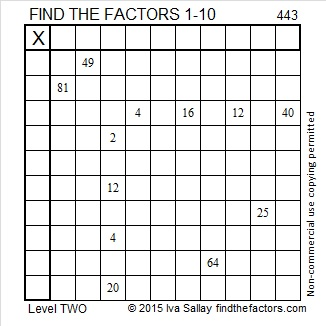# 443 and Level 2

Most people can tell just by looking that 443 cannot be evenly divided by 2, 3, or 5. Could it possibly be a prime number? Scroll down past the puzzle to know for sure.Print the puzzles or type the factors on this excel file: 10 Factors 2015-03-30

• 443 is a prime number.
• Prime factorization: 443 is prime.
• The exponent of prime number 443 is 1. Adding 1 to that exponent we get (1 + 1) = 2. Therefore 443 has exactly 2 factors.
• Factors of 443: 1, 443
• Factor pairs: 443 = 1 x 443
• 443 has no square factors that allow its square root to be simplified. √443 ≈ 21.0476How do we know that 443 is a prime number? If 443 were not a prime number, then it would be divisible by at least one prime number less than or equal to √443 ≈ 21.0476. Since 443 cannot be divided evenly by 2, 3, 5, 7, 11, 13, 17, or 19, we know that 443 is a prime number.This site uses Akismet to reduce spam. Learn how your comment data is processed.